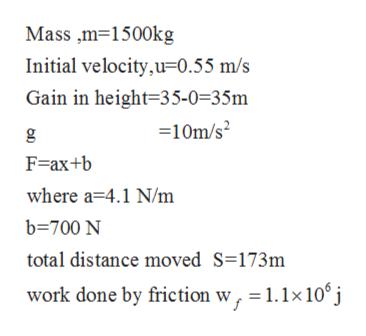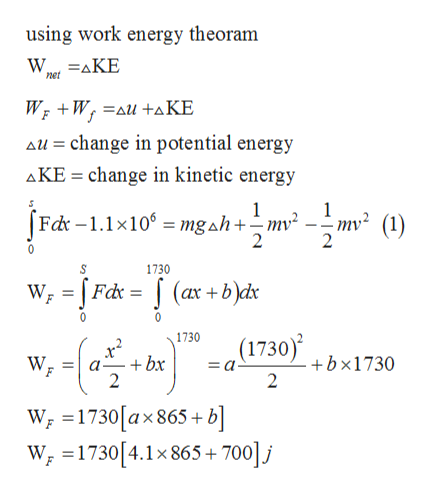# 3. 8.0 MJ of work is inputted to isothermally move a 1500 Kg mass from an elevation of zero to 35m and to change it velocity. The initial velocity of the mass is 0.55 m/s. The drag work for this process is described by a force which varies over the distance travel,F = ax + b; where F is in Newtons, the coefficient (a) has units of N/m and the constant (b) has units of N.For this application a = 4.1 N/m and b = 700 N. The force is applied over a distance of 1730 m. There is a heat loss from friction of 1.1(106) J during this process. Determine the final velocity of the mass.3b.  The input work for this process is from a combustion engine that has an efficiency of 0.28. The heating value of the fuel is 30.0 MJ/liters and its cost is \$0.83/liter. Determine the volume of fuel required and its cost to perform the process described in (3.).

Question

3. 8.0 MJ of work is inputted to isothermally move a 1500 Kg mass from an elevation of zero to 35m and to change it velocity. The initial velocity of the mass is 0.55 m/s. The drag work for this process is described by a force which varies over the distance travel,
F = ax + b; where F is in Newtons, the coefficient (a) has units of N/m and the constant (b) has units of N.
For this application a = 4.1 N/m and b = 700 N. The force is applied over a distance of 1730 m. There is a heat loss from friction of 1.1(106) J during this process. Determine the final velocity of the mass.
3b.  The input work for this process is from a combustion engine that has an efficiency of 0.28. The heating value of the fuel is 30.0 MJ/liters and its cost is \$0.83/liter. Determine the volume of fuel required and its cost to perform the process described in (3.).

check_circleExpert Solution
Step 1

Given data as per questionhelp_outlineImage TranscriptioncloseMass m 1500kg Initial velocity,u 0.55 m/s Gain in height 35-0-35m 10m/s2 F-ax+b where a 4.1 N/m b-700 N total distance moved S=173m work done by friction w, 1.1x10 j fullscreen
Step 2help_outlineImage Transcriptioncloseusing work energy theoram net Wr W au+aKE au change in potential energy change in kinetic energy AKE Fd -1.1x10mgah+mv -;mv (1) 1730 Fdc (ax+bdx 1730 (1730) +bx1730 2 W +bx = a a- F 2 W 1730[ax 865 + b] W =1730[4.1x 865+ 7001j fullscreen
Step 3

Using (1) and (2) an...

### Want to see the full answer?

See Solution

#### Want to see this answer and more?

Solutions are written by subject experts who are available 24/7. Questions are typically answered within 1 hour*

See Solution
*Response times may vary by subject and question
Tagged in

### Mechanical Engineering